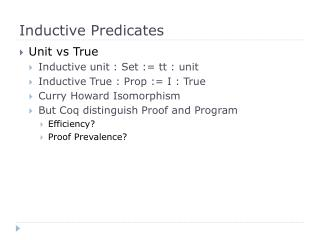DownloadDownload PresentationInductive Predicates

# Inductive Predicates

Download Presentation## Inductive Predicates

- - - - - - - - - - - - - - - - - - - - - - - - - - - E N D - - - - - - - - - - - - - - - - - - - - - - - - - - -
##### Presentation Transcript

1. Inductive Predicates • Unit vs True • Inductive unit : Set := tt : unit • Inductive True : Prop := I : True • Curry Howard Isomorphism • But Coq distinguish Proof and Program • Efficiency? • Proof Prevalence?

2. Propositional Logic • not = fun A : Prop => A -> False     : Prop -> Prop • Inductive and (A : Prop) (B : Prop) : Prop := conj : A -> B -> A /\ B • Inductive or (A : Prop) (B : Prop) : Prop :=    or_introl : A -> A \/ B | or_intror : B -> A \/ B

3. Bool Vs Prop • bool is datatype of two members, true, false • Prop is primitive type in Coq, and True, False are two members in Prop • Coq use Constructive Logic, while P\/~P is not allowed • Issues • bool is decidable • Constructive logic allow us to extract program from proof • We can write program by proof a theorem, though maybe less efficient

4. First-Order Logic • forall and exists • forall is built-in • exists: • Inductive ex (A : Type) (P : A -> Prop) : Prop :=    ex_intro : forall x : A, P x -> ex P

5. Tactics • Tauto • Solve intuition logic, only unfold not • Intuition • Use tauto, and apply tactics • Intuition=intuition auto. • Firstorder • Extend tauto to firstorder logic • Trivial • Restricted auto • auto • Eauto • Auto using eapply, generate ‘_’

6. Basic dependant type • Inductive isZero : nat -> Prop :=| IsZero : isZero 0. • Inductive even : nat -> Prop :=| EvenO : even O| EvenSS : forall n, even n -> even (S (S n)). • Example of product: forall a:A,B